Module 10 - Rules of Differentiation Introduction | Lesson 1 | Lesson 2 | Lesson 3 | Lesson 4 | Self-Test Lesson 10.2: The Product Rule In this lesson you will use an inductive method to develop the rule for the derivatives of products of functions. You will also learn how to save the commands in the History Area as a script. Because you want to save all the commands you enter during the course of this lesson, do not clear the History Area until the end of this lesson. Execute the NewProb command from the F6:Clean Up menu. Then clear the NewProb command from the History. Exploring the Derivative of xn sin x 10.2.1 Use your TI-89 to evaluate the derivatives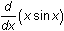,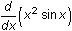, and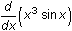. Click here for the answer. Predicting the Result and Testing 10.2.2 Predict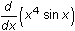then use your calculator to test your prediction. Click here for the answer. Generalizing the Results 10.2.3 Generalize the result by predicting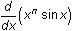and then test it with your TI-89. Click here for the answer. Finding Equivalent Expressions The TI-89 result probably looks different than your prediction. You can determine if the two expressions are equivalent by using the Expand feature of the Algebra menu. Clear the Edit Line by pressingPaste expand( into the Edit Line by selecting 3:expand( from the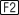:Algebra menu Highlight the last result shown in the History Area by pressingPaste the last result into the Edit Line by pressingEnter the closing parenthesis Evaluate the command by pressing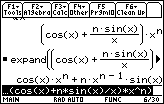Was this your prediction? Exploring the Derivative of Other Products To further explore the derivative of the product of two functions, see what happens if you replace xn in y = xn sin x with other functions and find their derivatives. 10.2.4 Find the derivatives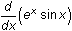and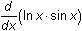by using the TI-89. Click here for the answers. Generalizing the Results In order to determine the pattern, it's helpful to recall that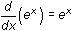and that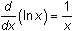. Both derivatives may be obtained by using the TI-89. 10.2.5 Predict the generalized derivative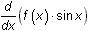and test your prediction with your TI-89. Click here to check your answer. Extending to Other Functions You have explored the derivative of the product of a general function and sin x. What happens if you replace sin x with other functions? 10.2.6 Predict the derivatives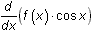and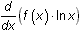. Click here for the answers. Writing the Theorem 10.2.7 Write a theorem that predicts the generalized derivative of the product of two functions,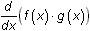. Then test your theorem with your TI-89. Click here for the answer. Saving Derivatives You have examined many derivatives to develop the Product Rule. You can save the commands used to find these derivatives in a script for future use. Open the Tools menu by pressingHighlight 2:Save Copy As by pressing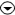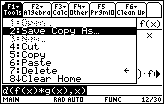Open the SAVE COPY AS dialog box by pressingMove the cursor to the Variable box by pressingYou will enter the name of the text file in this box. Enter the name "prodrule" in the Variable box You do not have to use the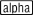key since the TI-89 is already in the alpha mode in this box.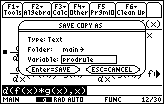Save the name by pressingClose the dialog box and save the file by pressingThe file you just created is called a script and it contains all the commands you entered in this lesson. You will use this script in Lesson 10.3. < Back | Next > ©Copyright 2007 All rights reserved. | Trademarks | Privacy Policy | Link Policy